# Data Preprocessing in Machine learning

In this page we will learn Data Preprocessing in Machine learning, What is Data Preprocessing in Machine learning?, Why do we need Data Preprocessing?, What is a CSV File in machine learning?, How to import Libraries, How to import the Datasets, What are the Ways to handle missing data?, Splitting the Dataset into the Training set and Test set, Feature Scaling.

## What is Data Preprocessing in Machine learning?

Data preprocessing is the procedure for preparing raw data for use in a machine learning model. It's the first and most important stage in building a machine learning model.

It is not always the case that we come across clean and prepared data when working on a machine learning project. And, before doing any data-related activity, it is necessary to clean the data and format it. As a result, we use a data pretreatment activity for this.

## Why do we need Data Preprocessing?

Real-world data sometimes contains noise, missing values, and is in an unsuitable format that cannot be used directly in machine learning models. Data preprocessing is a necessary task for cleaning data and making it suitable for a machine learning model, which improves the model's accuracy and efficiency.

The steps are as follows:

• Getting the dataset
• Importing libraries
• Importing datasets
• Finding Missing Data
• Encoding Categorical Data
• Splitting dataset into training and test set
• Feature scaling

## 1) Get the Dataset

The initial requirement for creating a machine learning model is a dataset, as a machine learning model is entirely based on data. The dataset is a collection of data in a certain format for a specific problem.

For example, if we want to construct a machine learning model for business objectives, the dataset will be different from the information necessary for a liver patient. As a result, each dataset is distinct from the others. We normally save the dataset as a CSV file so that we may use it in our programs. However, we may need to use an HTML or xlsx file on occasion.

## What is a CSV File?

CSV files, which stand for "Comma-Separated Values," are a file type that allows us to save tabular data, such as spreadsheets. It is suitable for large datasets and can be used in programs.

Here we will use a demo dataset for data preprocessing, and for practice, it can be downloaded from here, https://www.superdatascience.com/pages/machine-learning.

For real-world problems, we can download datasets online from various sources such as, https://www.kaggle.com/uciml/datasets,

https://archive.ics.uci.edu/ml/index.php etc.

We can also create our dataset by gathering data using various API with Python and put that data into a .csv file.

## 2) How to import Libraries?

We need to import several predefined Python libraries in order to execute data preparation with Python. These libraries are used for a variety of tasks. For data preprocessing, we will utilize the following three libraries:

Numpy: Numpy Python library is used to write code that includes any form of mathematical operation. It is the most important Python package for scientific calculations. Large, multidimensional arrays and matrices can also be added. As a result, we may import it in Python as:

``````
import numpy as np
```
```
Here we have used np, which is a short name for Numpy, and it will be used in the whole program.

Matplotlib: The second library is matplotlib, a Python 2D charting library that requires the import of a sub-library called pyplot. For the code, this library is used to plot any type of chart in Python. It will be imported in the following manner:

``````
import matplotlib.pyplot as mpt
```
```

Here we have used mpt as a short name for this library.

Pandas: The final library is Pandas, which is one of the most well-known Python libraries for importing and manipulating datasets. It's a data processing and analysis library that's free to use. It will be imported in the following manner:

``````
import pandas as pd
```
```

As a short name for this library, we've used pd. Consider the following illustration: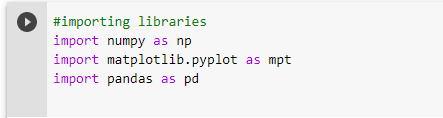## 3) How to import the Datasets

Now it's time to import the datasets we've gathered for our machine learning research. However, before we can import a dataset, we must make the current directory a working directory. The steps to create a working directory in Spyder IDE are as follows:

1. Make a copy of your Python file and place it in the dataset directory.
2. Select the desired directory using the File Explorer option in Spyder IDE.
3. It is a technology that is based on data.
4. To run the file, press F5 or choose the Run option.

[Note: the working directory can be any directory as long as it contains the relevant dataset. ]

The Python file, as well as the required dataset, may be seen in the image below. The current folder has now been designated as a working directory.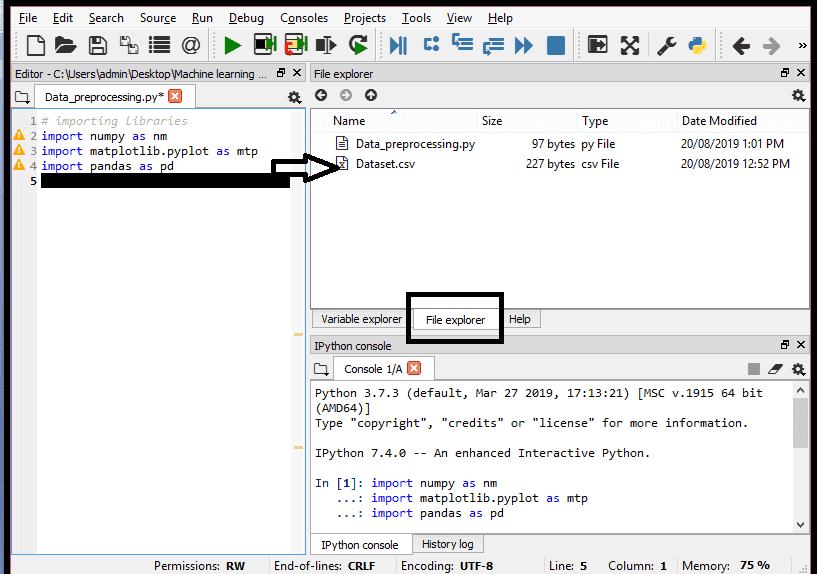read_csv() function: To import the dataset, we'll use the pandas library's read_csv() function, which reads a csv file and performs different actions on it. We may read a csv file locally as well as via a URL using this function.

We can use read_csv function as below:

``````
```
```

Here, data set is the name of the variable where we'll save our dataset, and we've supplied the name of our dataset into the method. When we run the above piece of code, the dataset will be successfully imported into our code. We can also inspect the imported data_set by double-clicking on data set in the section variable explorer. Consider the following illustration: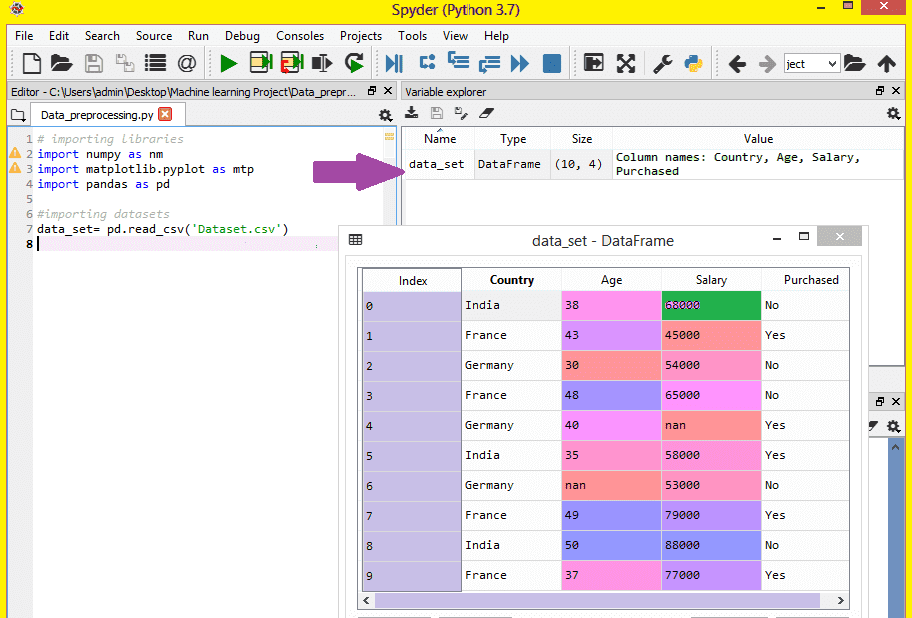Indexing begins at 0, which is the default indexing in Python, as shown in the accompanying figure. By selecting the format option, we may change the format of our dataset.

## Extracting dependent and independent variables:

In machine learning, it's critical to distinguish between the matrix of features (independent variables) and the dataset (dependent variables). Country, Age, and Salary are the three independent factors in our dataset, whereas Purchased is the only dependent variable.

## Extracting independent variable:

We'll utilize the Pandas library's iloc[ ] method to extract an independent variable. It's used to get the appropriate rows and columns out of a dataset.

``````
x = data_set.iloc[:,:-1].values
```
```

The first colon(:) is used to take all the rows, and the second colon(:) is used to take all the columns in the above code. In this case, we've used: We don't want to take the last column because it contains the dependant variable, therefore we'll use: -1. As a result, we will obtain the feature matrix.

We will receive the following output if we run the above code:
``````
[['India' 38.0 68000.0]
['France' 43.0 45000.0]
['Germany' 30.0 54000.0]
['France' 48.0 65000.0]
['Germany' 40.0 nan]
['India' 35.0 58000.0]
['Germany' nan 53000.0]
['France' 49.0 79000.0]
['India' 50.0 88000.0]
['France' 37.0 77000.0]]
```
```

As we can see in the above output, there are only three variables.

## Extracting dependent variable:

To extract dependent variables, again, we will use Pandas .iloc[] method.

``````
y = data_set.iloc[:,3].values
```
```

Here we have taken all the rows with the last column only. It will give the array of dependent variables.

By executing the above code, we will get output as:

## Output:

``````
array(['No', 'Yes', 'No', 'No', 'Yes', 'Yes', 'No', 'Yes', 'No', 'Yes'], dtype=object)
```
```

[Note: extraction is essential if you're using Python for machine learning, but it's not required if you're using R.]

## 4) Handling Missing data:

The following phase in the data preparation process is to deal with missing data in the datasets. If some of the data in our dataset is missing, it could be a significant challenge for our machine learning model. As a result, handling missing values in the dataset is required.

## What are the ways to handle missing data?

There are primarily two approaches to dealing with missing data:

By deleting a specific row: The first method is typically used to deal with null data. We just delete the null values from the specific row or column in this manner. However, this method is inefficient, and eliminating data may result in the loss of information, resulting in an inaccurate output.

By calculating the mean: In this case, we'll compute the mean of the column or row that contains any empty values and use it to fill in the blanks. This method works well for characteristics that contain quantitative data, such as age, salary, year, and so on. We'll take this technique in this case.

We will utilize the Scikit-learn package in our code to handle missing values, which offers numerous tools for developing machine learning models. The Imputer class from the sklearn.preprocessing library will be used here. The code for it is as follows:

``````
#handling missing data (Replacing missing data with the mean value)
from sklearn.preprocessing import Imputer
imputer = Imputer(missing_values ='NaN', strategy='mean', axis = 0)

#Fitting imputer object to the independent variables x.
imputerimputer = imputer.fit(x[:, 1:3])

#Replacing missing data with the calculated mean value
x[:, 1:3] = imputer.transform(x[:, 1:3])
```
```

### Output:

``````
array([['India', 38.0, 68000.0],
['France', 43.0, 45000.0],
['Germany', 30.0, 54000.0],
['France', 48.0, 65000.0],
['Germany', 40.0, 65222.22222222222],
['India', 35.0, 58000.0],
['Germany', 41.111111111111114, 53000.0],
['France', 49.0, 79000.0],
['India', 50.0, 88000.0],
['France', 37.0, 77000.0]], dtype=object
```
```

## 5) Encoding Categorical data:

Categorical data is information that is divided into categories. For example, there are two categorical variables in our dataset: Country and Purchased.

Because a machine learning model is entirely based on mathematics and numbers, having a categorical variable in our dataset could cause problems while developing the model. As a result, these category variables must be converted to integers.

For the Country variable:

We'll start by converting the nation variables into categorical data. So, we'll use the LabelEncoder() class from the preprocessing library to accomplish this.

``````
#Catgorical data
#for Country Variable
from sklearn.preprocessing import LabelEncoder
label_encoder_x = LabelEncoder()
x[:, 0] = label_encoder_x.fit_transform(x[:, 0])
```
```

### Output:

``````
Out:
array([[2, 38.0, 68000.0],
[0, 43.0, 45000.0],
[1, 30.0, 54000.0],
[0, 48.0, 65000.0],
[1, 40.0, 65222.22222222222],
[2, 35.0, 58000.0],
[1, 41.111111111111114, 53000.0],
[0, 49.0, 79000.0],
[2, 50.0, 88000.0],
[0, 37.0, 77000.0]], dtype=object)
```
```

## Explanation:

The sklearn library's LabelEncoder class was imported in the above code. The variables have been correctly encoded into digits by this class.

However, there are three nation variables in our situation, and as we can see from the result above, these variables are encoded as 0, 1, and 2. The machine learning model may believe that there is some link between these variables based on these values, resulting in the incorrect output. To solve this problem, we'll employ dummy encoding.

## Dummy Variables:

Variables with values of 0 or 1 are referred to as dummy variables. The presence of a variable in a certain column is indicated by a 1 value, while the rest of the variables are assigned a value of 0. We'll have a number of columns equal to the number of categories if we use dummy encoding.

Because we have three categories in our dataset, it will generate three columns with 0 and 1 values. We'll utilize the preprocessing library's OneHotEncoder class for Dummy Encoding.

``````
#for Country Variable
from sklearn.preprocessing import LabelEncoder, OneHotEncoder
label_encoder_x = LabelEncoder()
x[:, 0] = label_encoder_x.fit_transform(x[:, 0])
#Encoding for dummy variables
onehot_encoder = OneHotEncoder(categorical_features= )
x = onehot_encoder.fit_transform(x).toarray()
```
```

### Output:

``````
array([[0.00000000e+00, 0.00000000e+00, 1.00000000e+00, 3.80000000e+01, 6.80000000e+04],
[1.00000000e+00, 0.00000000e+00, 0.00000000e+00, 4.30000000e+01, 4.50000000e+04],
[0.00000000e+00, 1.00000000e+00, 0.00000000e+00, 3.00000000e+01, 5.40000000e+04],
[1.00000000e+00, 0.00000000e+00, 0.00000000e+00, 4.80000000e+01, 6.50000000e+04],
[0.00000000e+00, 1.00000000e+00, 0.00000000e+00, 4.00000000e+01, 6.52222222e+04],
[0.00000000e+00, 0.00000000e+00, 1.00000000e+00, 3.50000000e+01, 5.80000000e+04],
[0.00000000e+00, 1.00000000e+00, 0.00000000e+00, 4.11111111e+01, 5.30000000e+04],
[1.00000000e+00, 0.00000000e+00, 0.00000000e+00, 4.90000000e+01, 7.90000000e+04],
[0.00000000e+00, 0.00000000e+00, 1.00000000e+00, 5.00000000e+01, 8.80000000e+04],
[1.00000000e+00, 0.00000000e+00, 0.00000000e+00, 3.70000000e+01, 7.70000000e+04]])
```
```

As we can see in the above output, all the variables are encoded into numbers 0 and 1 and divided into three columns.
It can be seen more clearly in the variables explorer section, by clicking on x option as: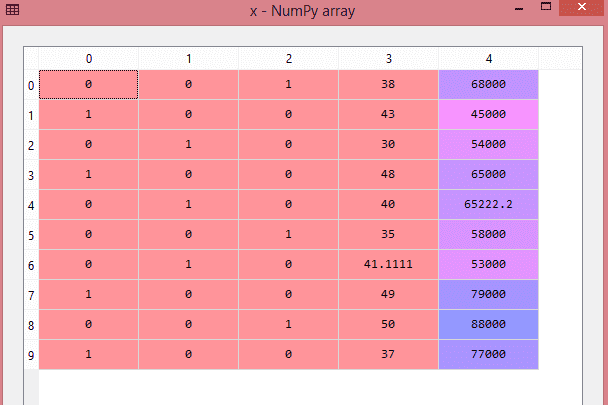## For Purchased Variable:

``````
labelencoder_y = LabelEncoder()
y = labelencoder_y.fit_transform(y)
```
```

### Output:

``````
Out: array([0, 1, 0, 0, 1, 1, 0, 1, 0, 1])
```
```

It can also be seen as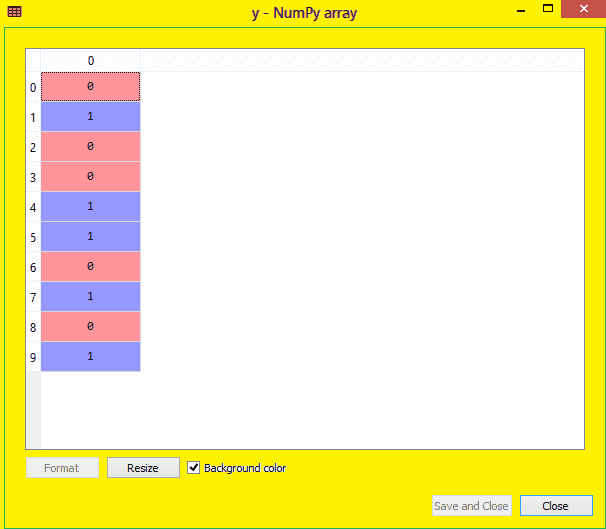## 6) Splitting the Dataset into the Training set and Test set

We divide our dataset into a training set and a test set in machine learning data preprocessing. This is an important step in data preprocessing since it improves the performance of our machine learning model.

Assume we've trained our machine learning model with one dataset and then tested it with a different dataset. Then our model will have trouble understanding the relationships between the models.

If we train our model properly and its training accuracy is high, but then give it a fresh dataset, the model's performance will suffer. As a result, we always strive to create a machine learning model that works well with both the training and test datasets. These datasets can be defined as follows: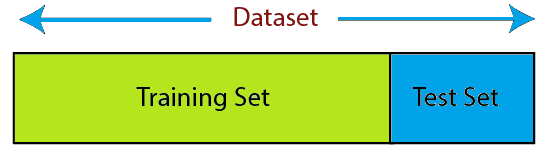Training Set: The training set is a subset of the dataset used to train the machine learning model, and the output is already known.

Test set: is a subset of the dataset used to test the machine learning model, and the model predicts the output using the test set.

We'll use the lines of code below to partition the dataset.

``````
from sklearn.model_selection import train_test_split
x_train, x_test, y_train, y_test = train_test_split(x, y, test_size = 0.2, random_state = 0)
```
```

### Explanation:

• The first line in the code above is used to split the dataset's arrays into random train and test sections.
• In the second line, we have used four variables for our output that are
• x_train: features for the training data
• x_test: features for testing data
• y_train: Dependent variables for training data
• y_test: Independent variable for testing data
• We gave four parameters to the train_test split() function, the first two of which are arrays of data and test_size, which specifies the test_size. The test size value could be.5,.3, or.2, indicating the training and testing sets' dividing ratio.
• The final option, random state, is used to seed a random generator so that you always obtain the same output, and the most common value is 42.

### Output:

We will obtain four different variables if we run the preceding code, which can be seen in the variable explorer section.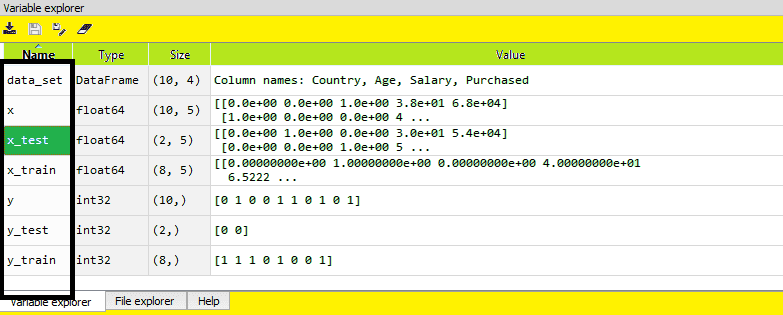As we can see in the above image, the x and y variables are divided into 4 different variables with corresponding values.

## 7) Feature Scaling

As we can see, the age and salary column values are not on the same scale. A machine learning model is based on Euclidean distance, and if we do not scale the variable, then it will cause some issue in our machine learning model.

Euclidean distance is given as: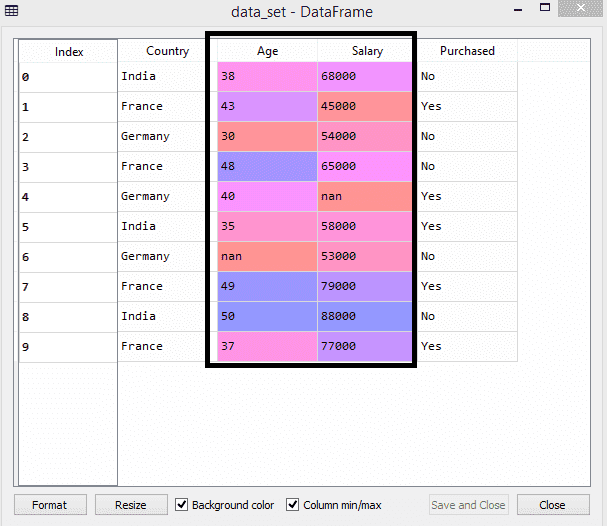As can be seen, the values in the age and salary columns are not on the same scale. A machine learning model is built on Euclidean distance, and if we don't scale the variable, our machine learning model will have issues.

The Euclidean distance is calculated as follows: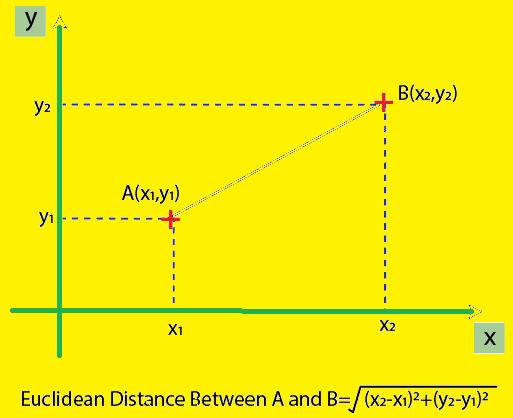If we compute any two numbers from age and salary, the salary values will always outnumber the age values, resulting in an inaccurate result. To solve this problem, we must use feature scaling in machine learning.

In machine learning, there are two approaches to achieve feature scaling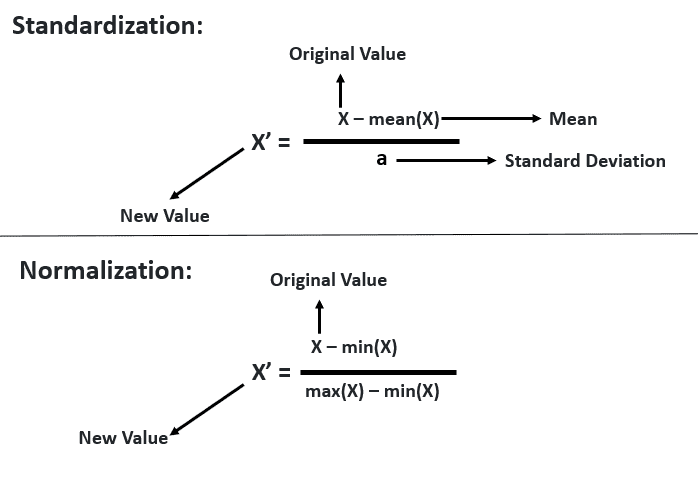Here, we will use the standardization method for our dataset.
For feature scaling, we will import StandardScaler class of sklearn.preprocessing library as:

``````
from sklearn.preprocessing import StandardScaler
```
```

Now, we will create the object of StandardScaler class for independent variables or features. And then we will fit and transform the training dataset.

``````
st_x = StandardScaler()
x_train = st_x.fit_transform(x_train)
```
```

For test dataset, we will directly apply transform() function instead of fit_transform() because it is already done in training set.

``````
x_test = st_x.transform(x_test)
```
```

## Output:

By executing the above lines of code, we will get the scaled values for x_train and x_test as: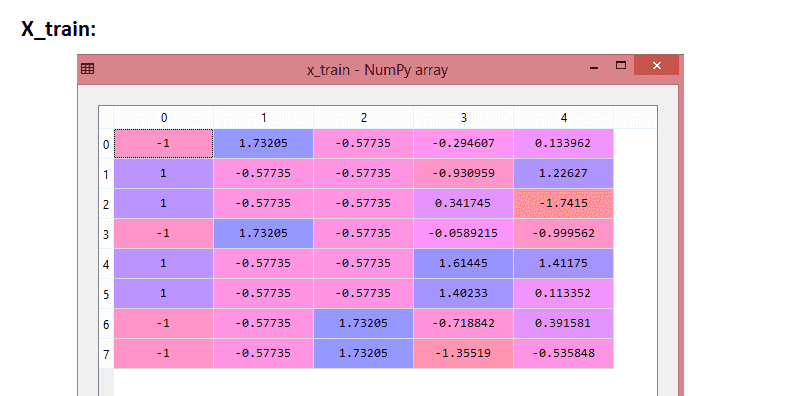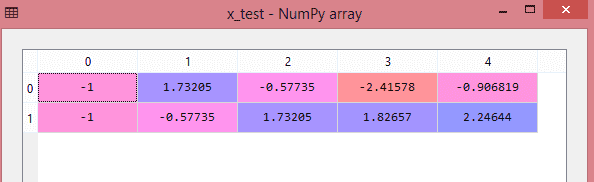All of the variables are scaled between -1 and 1 in the above output, as can be seen.

Note: the dependent variable has not been scaled because there are only two possible values: 0 and 1. However, if these variables have a wider range of values, we will need to scale them as well.

Combining all the steps:

``````
#importing libraries
import numpy as nm
import matplotlib.pyplot as mtp
import pandas as pd

#importing datasets

#Extracting Independent Variable
x= data_set.iloc[:, :-1].values

#Extracting Dependent variable
y= data_set.iloc[:, 3].values

#handling missing data(Replacing missing data with the mean value)
from sklearn.preprocessing import Imputer
imputer= Imputer(missing_values ='NaN', strategy='mean', axis = 0)

#Fitting imputer object to the independent varibles x.
imputerimputer= imputer.fit(x[:, 1:3])

#Replacing missing data with the calculated mean value
x[:, 1:3]= imputer.transform(x[:, 1:3])

#for Country Variable
from sklearn.preprocessing import LabelEncoder, OneHotEncoder
label_encoder_x= LabelEncoder()
x[:, 0]= label_encoder_x.fit_transform(x[:, 0])

#Encoding for dummy variables
onehot_encoder= OneHotEncoder(categorical_features= )
x= onehot_encoder.fit_transform(x).toarray()

#encoding for purchased variable
labelencoder_y= LabelEncoder()
y= labelencoder_y.fit_transform(y)

# Splitting the dataset into training and test set.
from sklearn.model_selection import train_test_split
x_train, x_test, y_train, y_test= train_test_split(x, y,test_size= 0.2, random_state=0)

#Feature Scaling of datasets
from sklearn.preprocessing import StandardScaler
st_x= StandardScaler()
x_train= st_x.fit_transform(x_train)
x_test= st_x.transform(x_test)
```
```

All of the data preprocessing procedures are combined in the above code. Some stages or lines of code, however, are not required for every machine learning models. As a result, we may remove them from our code and reuse it across all models.